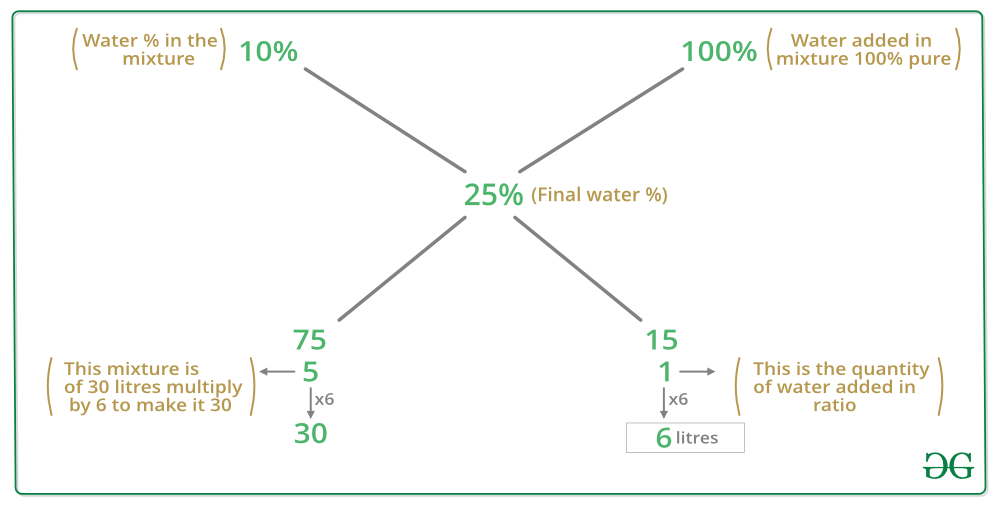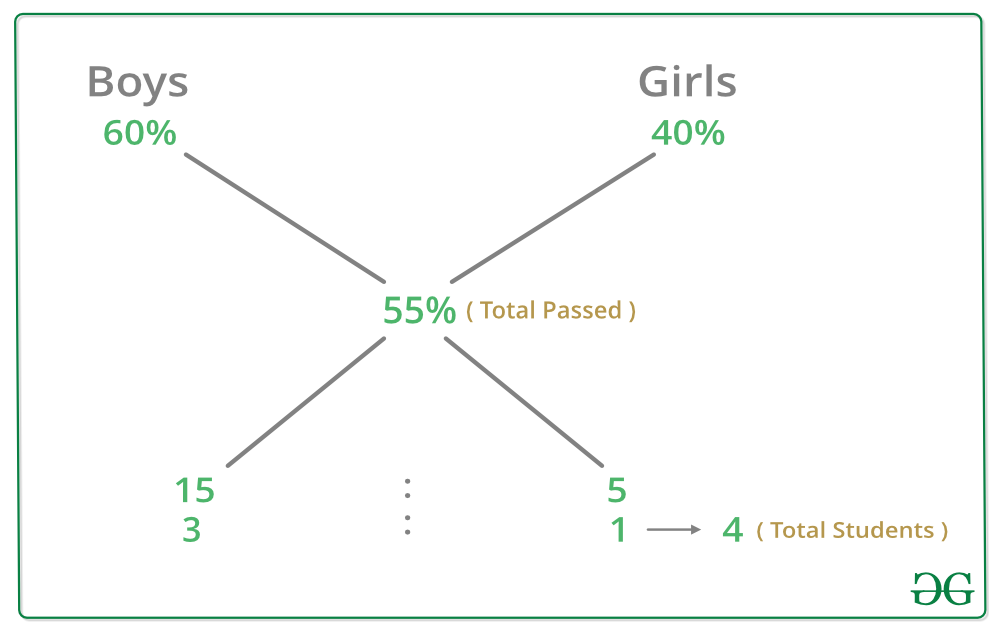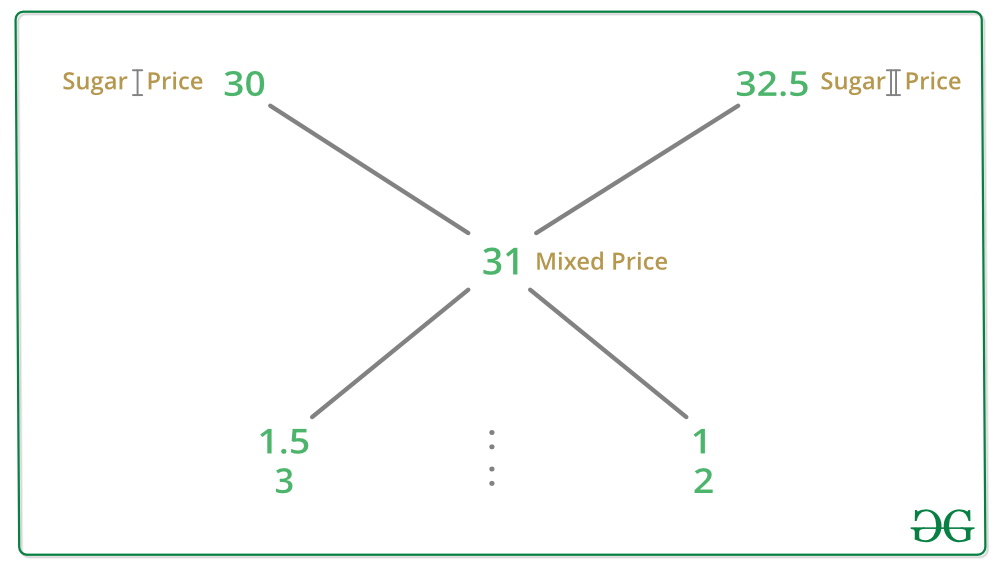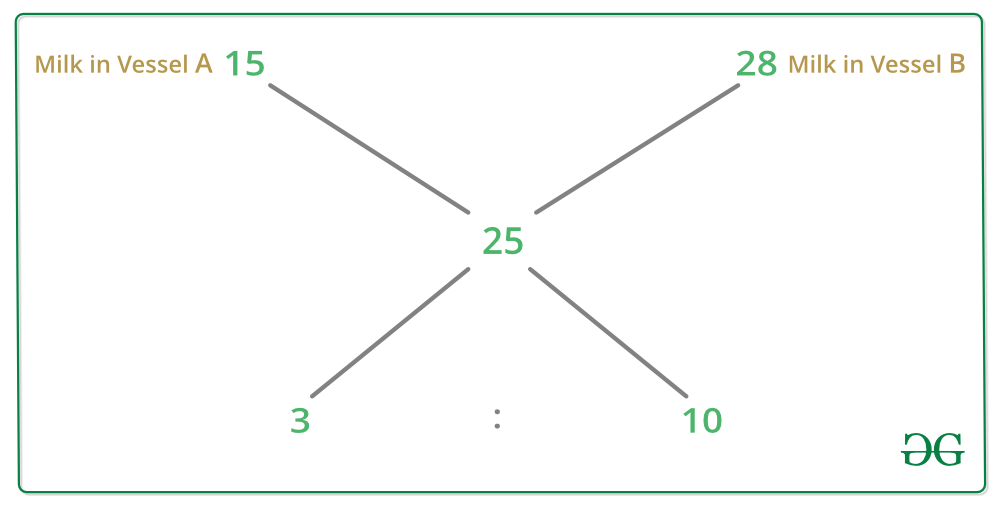Related Articles
• Explore Our Geeks Community
• SBI PO Quantitative Aptitude 2022
• Special Series - Sequences and Series | Class 11 Maths

# Mixture and Alligation | Set 2

Question 1: 30 litres of a mixture of milk and water contains 10% of water, the water to be added, to make the water content 25% in the new mixture. Find how many litres water will be added?
Solution : Water in the 30 litre of mixture = 30 x 10/100 = 3 litres
Milk in the mixture = 30 – 3 = 27 litres
Let x litres of water is mixed.
Acc. to question
(3 + x)/(30 + x) = 25/100
4(3 + x) = 30 + x
12 + 4x = 30 + x
3x = 18
x = 6
Hence, 6 litres of water to be added in the mixture.

Alternate :Question 2: 25000 students appeared in an exam. 60% of the boys and 40% of the girls cleared the examination. If the total percent of students qualifying is 55%, how many girls appeared in the exam?
Solution : By alligation method4 -> 25000
1 -> 6250
Hence, number of girls passed in the exam is 6250.

Question 3: In what ratio must a shopkeeper mix sugar at Rs 30/kg and Rs 32.5/kg, so that by selling the mixture at Rs 34.1/kg he may gain 10%.
Solution : Acc. to question
SP of 1 kg of mixture = Rs 34.1
Profit = 10% = 10/100 = 1/10
SP = 1 + 10 = 11 unit
11 units ->34.1
1 unit -> 3.1
10 unit -> 31
We obtain the CP of the mixture Rs 31.
To obtain the ratio use alligation methodHence, the ratio in which he mixed is 3:2

Question 4: Two vessels A and B contain mixture of milk and water in ratios 3:4 and 4:1 respectively. In what ratio should quantities of mixture be taken from A and B to form a mixture in which milk to water is in the ratio 5:2?
Solution :Acc. to question

```                   Milk        Water        total
Mixture A          3x5     :    4x5      ->    7       LCM(7, 5, 7)=35
Mixture B          4x7     :    1x7      ->    5
Final mixture      5x5     :    2x5      ->    7```

To make quantity equal of both multiply with 7 and 5 respectively.

```                   Milk       Water
Mixture A          15     :    20
Mixture B          28     :    7
Final mixture      25     :    10    ```

To obtain the ratio in which A and B mixed we apply alligation ruleHence, the required ratio is 3:10

Question 5: 40 kg of an alloy mixed with 100 kg of alloy B. If alloy A has lead and copper in the ratio 3:2 and alloy B has copper and tin in the ratio 1:3., then the amount of copper in the new alloy is
Solution :Alloy A 40 kg contains lead and copper in ratio 3:2
So, 3+2 = 5
5 unit -> 40
1 unit -> 8
2 unit -> 16
Amount of copper in alloy A is 16 kg.
Alloy B contains copper and tin in ratio 1:3
So, 1+3 = 4
4 unit -> 100
1 -> 25
Amount of copper in alloy B is 25 kg
Hence, amount of copper in the new alloy is 16 + 25 = 41 kg.

Whether you're preparing for your first job interview or aiming to upskill in this ever-evolving tech landscape, GeeksforGeeks Courses are your key to success. We provide top-quality content at affordable prices, all geared towards accelerating your growth in a time-bound manner. Join the millions we've already empowered, and we're here to do the same for you. Don't miss out - check it out now!

Previous
Next